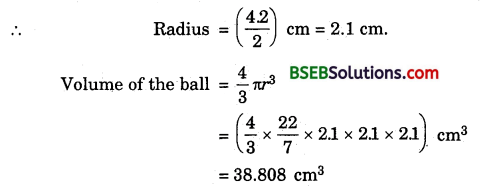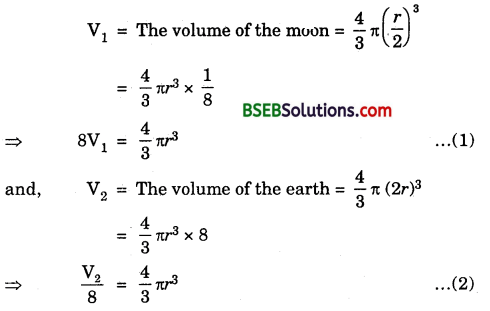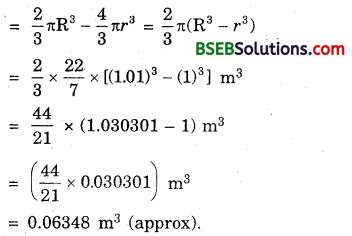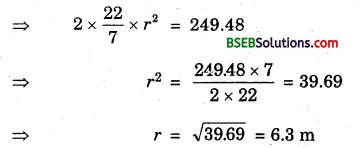# HSSlive: Plus One & Plus Two Notes & Solutions for Kerala State Board

## BSEB Class 9 Maths Chapter 13 Surface Areas and Volumes Ex 13.8 Textbook Solutions PDF: Download Bihar Board STD 9th Maths Chapter 13 Surface Areas and Volumes Ex 13.8 Book AnswersBSEB Class 9 Maths Chapter 13 Surface Areas and Volumes Ex 13.8 Textbook Solutions PDF: Download Bihar Board STD 9th Maths Chapter 13 Surface Areas and Volumes Ex 13.8 Book Answers

BSEB Class 9th Maths Chapter 13 Surface Areas and Volumes Ex 13.8 Textbooks Solutions and answers for students are now available in pdf format. Bihar Board Class 9th Maths Chapter 13 Surface Areas and Volumes Ex 13.8 Book answers and solutions are one of the most important study materials for any student. The Bihar Board Class 9th Maths Chapter 13 Surface Areas and Volumes Ex 13.8 books are published by the Bihar Board Publishers. These Bihar Board Class 9th Maths Chapter 13 Surface Areas and Volumes Ex 13.8 textbooks are prepared by a group of expert faculty members. Students can download these BSEB STD 9th Maths Chapter 13 Surface Areas and Volumes Ex 13.8 book solutions pdf online from this page.

## Bihar Board Class 9th Maths Chapter 13 Surface Areas and Volumes Ex 13.8 Books Solutions

 Board BSEB Materials Textbook Solutions/Guide Format DOC/PDF Class 9th Subject Maths Chapter 13 Surface Areas and Volumes Ex 13.8 Chapters All Provider Hsslive

## How to download Bihar Board Class 9th Maths Chapter 13 Surface Areas and Volumes Ex 13.8 Textbook Solutions Answers PDF Online?

2. Click on the Bihar Board Class 9th Maths Chapter 13 Surface Areas and Volumes Ex 13.8 Answers.
3. Look for your Bihar Board STD 9th Maths Chapter 13 Surface Areas and Volumes Ex 13.8 Textbooks PDF.
4. Now download or read the Bihar Board Class 9th Maths Chapter 13 Surface Areas and Volumes Ex 13.8 Textbook Solutions for PDF Free.

## BSEB Class 9th Maths Chapter 13 Surface Areas and Volumes Ex 13.8 Textbooks Solutions with Answer PDF Download

Find below the list of all BSEB Class 9th Maths Chapter 13 Surface Areas and Volumes Ex 13.8 Textbook Solutions for PDF’s for you to download and prepare for the upcoming exams:

## BSEB Bihar Board Class 9th Maths Solutions Chapter 13 Surface Areas and Volumes Ex 13.8

Question 1.
Find the volume of a sphere whose radius is
(i) 7 cm
(ii) 0.63 m
Solution:
(i) We have : r = radius of the sphere = 7 cm.(ii) We have : r = radius of the sphere = 0.63 mQuestion 2.
Find the amount of water displaced by a solid spherical ball of diameter
(i) 28 cm
(ii) 0.21 m
Solution:
(i) Diameter of the spherical ball = 28 cm
∴ Radius = 282 cm = 14 cm
Amount of water displaced by the spherical ball
= Its volume = 43 πr³
= (43 x 227 x 14 x 14 x 14) cm³
= 344963 cm³
= 1149823 cm³
= 1149823 cm³.

(ii) Diameter of the spherical ball = 0.21 m
∴ Radius = (0.212) = 0.105 m
Amount of water displaced by the spherical ball
= Its volume = 43 πr³
= (43 x 227 x 0.105 x 0105 x 0105) m³
= 0.004851 m³.

Question 3.
The diameter of a metallic ball is 4.2 cm. What is the mass of the ball, if the density of the metal is 8.9 g per cm³?
Solution:
Diameter of the ball = 4.2 cmDensity of the metal is 8.9 g per cm³
Mass of the ball = (38.808 x 8.9) g = 345.3912 g.

Question 4.
The diameter of the moon is approximately one- fourth the diameter of the earth. What fraction of the volume of the earth is the volume of the moon?
Solution:
Let the diameter of the moon be r. Then, the radius of the moon = 𝑟2
According to the question, diameter of the earth is 4r, so 4 r its radius = 4𝑟2 = 2rHence, the volume of the earth is 164 of the volume of the earth.

Question 5.
How many litres of milk can a hemispherical bowl of diameter 10.5 cm hold?
Solution:
Diameter of a hemispherical bowl = 10.5 cm.Hence, the hemispherical bowl can hold 303 l (approx.) of milk.

Question 6.
A hemispherical tank is made up of an iron sheet 1 cm thick. If the inner radius is 1 m, then find the volume of the iron used to make the tank.
Solution:
Let R cm and r cm be respectively the external and internal radii of the hemispherical vessel. Then,
R = 1.01 (as thickness = 1 cm = .01 m)
and r = 1 m.
Volume of iron used = External volume – Internal volumeQuestion 7.
Find the volume of a sphere whose surface area is 154 cm².
Solution:
Let r cm be the radius of the sphere.
So, surface area = 154 cm²Question 8.
A dome of a building is in the form of a hemisphere. From inside, it was white-washed at the cost of Rs 498.96. If the cost of white-washing is Rs 2.00 per square metre, find the
(i) inside surface area of the dome.
(ii) volume of the air inside the dome.
Solution:
(i) Inside surface area of the dome(ii) Let r be the radius of the dome.
∴ Surface area = 2πr²Volume of the air inside the dome = Volume of the dome
= 23 πr³
= 23 x 227 x 6.3 x 6.3 x 6.3 m³
= 523.9 m³ (approx).

Question 9.
Twenty seven solid iron spheres, each of radius r and surface area S are melted to form a sphere with surface area S’, Find the
(i) radius r’ of the new sphere,
(ii) ratio of S and S’.
Solution:
(i) Volume of 27 solid sphere of radius
r = 27 x 43πr³ … (1)
Volume of the new sphere of radius r’ = 43 πr’³ … (2)
According to the problem, we have
43πr’³ = 27 x 43πr³
⇒ r’3 = 27r³ = (3r)³
∴ r’ = 3r.

(ii) Required ratio = 𝑆𝑆′ = 4𝜋𝑟24𝜋𝑟22 = 𝑟2(3𝑟)2
= 𝑟29𝑟2 = 19 = 1 : 9.

Question 10.
A capsule of medicine is in the shape of a sphere of diameter 3.5 mm. How much medicine (in mm³) is needed to fill this capsule?
Solution:
Diameter of the spherical capsule = 3.5 mm
= 1.75 mm.
Medicine needed for its filling = Volume of spherical capsule
= 43πr³
= (43 x 227 x 1.75 x 1.75 x 1.75) m³
= 22.46 m³ (approx).

## Bihar Board Class 9th Maths Chapter 13 Surface Areas and Volumes Ex 13.8 Textbooks for Exam Preparations

Bihar Board Class 9th Maths Chapter 13 Surface Areas and Volumes Ex 13.8 Textbook Solutions can be of great help in your Bihar Board Class 9th Maths Chapter 13 Surface Areas and Volumes Ex 13.8 exam preparation. The BSEB STD 9th Maths Chapter 13 Surface Areas and Volumes Ex 13.8 Textbooks study material, used with the English medium textbooks, can help you complete the entire Class 9th Maths Chapter 13 Surface Areas and Volumes Ex 13.8 Books State Board syllabus with maximum efficiency.

## FAQs Regarding Bihar Board Class 9th Maths Chapter 13 Surface Areas and Volumes Ex 13.8 Textbook Solutions

#### How to get BSEB Class 9th Maths Chapter 13 Surface Areas and Volumes Ex 13.8 Textbook Answers??

Students can download the Bihar Board Class 9 Maths Chapter 13 Surface Areas and Volumes Ex 13.8 Answers PDF from the links provided above.

#### Can we get a Bihar Board Book PDF for all Classes?

Yes you can get Bihar Board Text Book PDF for all classes using the links provided in the above article.

## Important Terms

Bihar Board Class 9th Maths Chapter 13 Surface Areas and Volumes Ex 13.8, BSEB Class 9th Maths Chapter 13 Surface Areas and Volumes Ex 13.8 Textbooks, Bihar Board Class 9th Maths Chapter 13 Surface Areas and Volumes Ex 13.8, Bihar Board Class 9th Maths Chapter 13 Surface Areas and Volumes Ex 13.8 Textbook solutions, BSEB Class 9th Maths Chapter 13 Surface Areas and Volumes Ex 13.8 Textbooks Solutions, Bihar Board STD 9th Maths Chapter 13 Surface Areas and Volumes Ex 13.8, BSEB STD 9th Maths Chapter 13 Surface Areas and Volumes Ex 13.8 Textbooks, Bihar Board STD 9th Maths Chapter 13 Surface Areas and Volumes Ex 13.8, Bihar Board STD 9th Maths Chapter 13 Surface Areas and Volumes Ex 13.8 Textbook solutions, BSEB STD 9th Maths Chapter 13 Surface Areas and Volumes Ex 13.8 Textbooks Solutions,
Share: